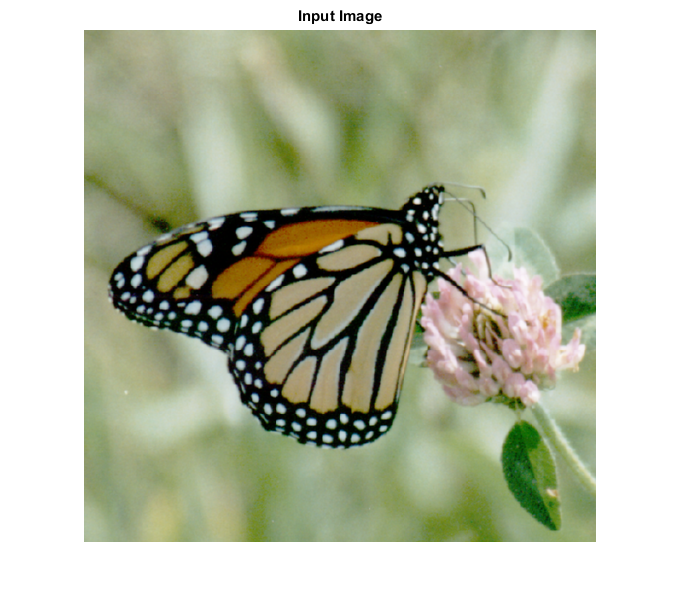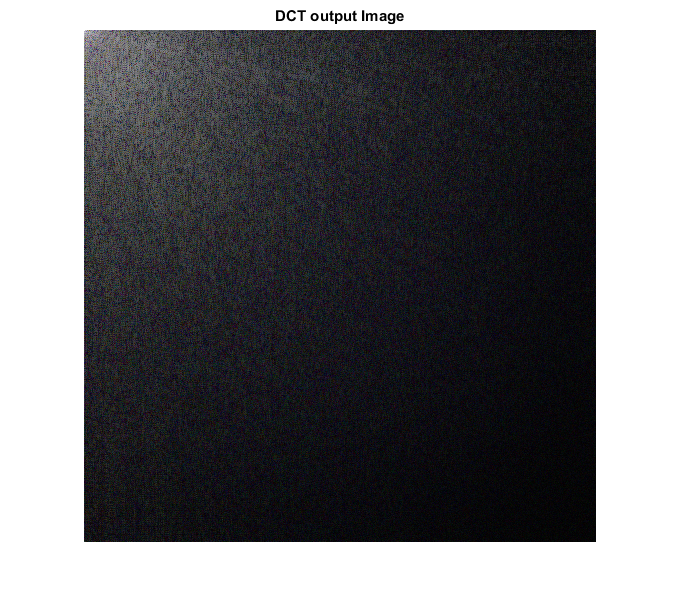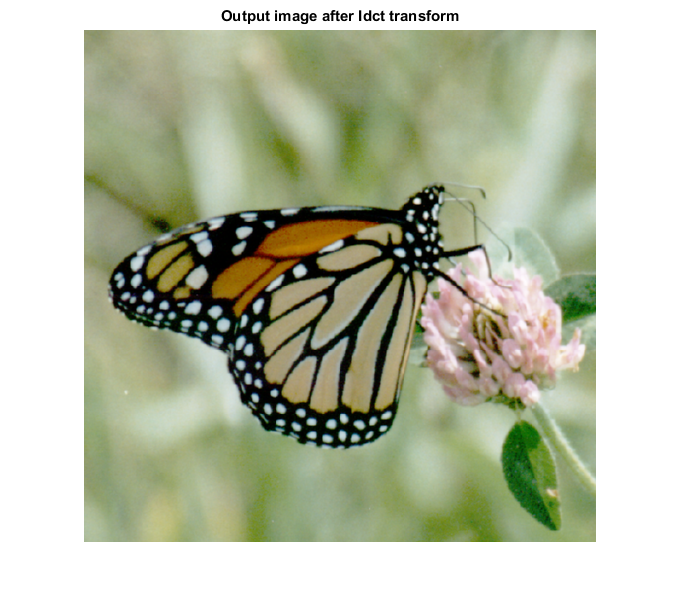# IDCT_CVIP

idct_cvip() - perform inverse discrete cosine transform.

## SYNTAX

`img = idct_cvip(input_spect,block_size)`

Input Parameters include :

• input_spect - The DCT spectrum of an image. This spectrum must be obtained by dct_cvip().
• block_size - The block_size shouls be same as the block_size used for forward DCT .

Output Parameters include :

• Spect - The inverse Discrete cosine transform of the input spectrum.

## DESCRIPTION

This function computes the inverse discrete cosine transform of the input image. The argument block_size should match the same value given for the forward transform. For more details look at the reference below.

## REFERENCE

1. Scott E Umbaugh. DIGITAL IMAGE PROCESSING AND ANALYSIS: Applications with MATLAB and CVIPtools, 3rd Edition.

## EXAMPLE

```% Read Image

% Calling  DCT function

spect = dct_cvip( I,[] );

% Calling inverse DCT function

I_hat = idct_cvip( spect,[] );

% Display input image

figure;imshow(I);title('Input Image');

% Display output images

figure;imshow(remap_cvip(log(1+abs(spect))));title('DCT output Image');

figure; imshow(hist_stretch_cvip(I_hat,0,1,0,0));title('Output image after Idct transform');
```